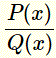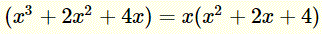# Partial Fractions Decompositions

Tutorial on decomposing complicated fractions into simpler manageable fractions. One of its important applications is in Integration Using Partial Fractions in calculus.

## Rules of Decomposition into Partial Fractions

How to decompose a rational functioninto partial fractions?

1 - Factor completely polynomial Q(x) in denominator into factors of the formExample
LetThe denominator is factored as follows2 + 2 x + 4 is irreducible (cannot be factored) over the real.

2 - For each factor of the form $(ax + b)^m$, the decomposition includes the following sum of fractions
$\dfrac{C1}{ax + b}+\dfrac{C2}{(ax + b)^2}+...+\dfrac{Cm}{(ax + b)^m}$

Example
The fraction $\dfrac{2}{(x-2)^3}$ is decomposed as
$\dfrac{2}{(x-2)^3}=\dfrac{C1}{x-2}+\dfrac{C2}{(x-2)^2}+\dfrac{C3}{(x-2)^3}$
3 - For each factor of the form $(a x^2 + b x + c)^n$, the decomposition includes the following sum of fractions
$\dfrac{A1 x + B1}{a x^2 + b x + c} + \dfrac{A2 x + B2}{(a x^2 + b x + c)^2} + ... + \dfrac{An x + Bn}{a x^2 + b x + c)^n}$

## Examples with Detailed Solutions

### Example 1

Decompose into partial fractions

$\dfrac{2 x + 5}{x^2-x-2}$

Solution to Example 1:
We start by factoring the denominator
$x^2 - x - 2 = (x - 2)(x + 1)$
Both factors are linear, hence the given fraction is decomposed as follows

$\dfrac{2 x + 5}{x^2-x-2}=\dfrac{A}{x-2}+\dfrac{B}{x+1}$

Multiply both side of the above equation by the least common denominator, $(x - 2)(x + 1)$, and simplify to obtain an equation of the form
$2 x+5 = A(x + 1) + B(x - 2)$
Expand the right side and group like terms
$2 x + 5 = x (A + B) + A - 2 B$
For the right and left polynomials to be equal we need to have
$2 = A + B$ and $5 = A - 2 B$
Solve the above system to obtain
$A = 3$ and $B = -1$
Substitute $A$ and $B$ in the suggested decomposition above to obtain
$\dfrac{2 x + 5}{x^2-x-2}=\dfrac{3}{x-2}-\dfrac{1}{x+1}$

As an exercise, group terms on the right to obtain the left side

### Example 2

Decompose into partial fractions
$\dfrac{1-2 x}{x^2+2x+1}$

Solution to Example 2:
We start by factoring the denominator
$x^2 + 2 x + 1 = (x + 1)^2$
Using the rule above, the given fraction is decomposed as follows

$\dfrac{1-2 x}{x^2+2x+1}=\dfrac{A}{x+1}+\dfrac{B}{(x+1)^2}$

Multiply both side of the above equation by $(x + 1)^2$, and simplify to obtain an equation of the form
$1 - 2 x = A(x + 1) + B$
Expand the right side and group like terms
$-2x + 1 = A x + (A + B)$
For the right and left polynomials to be equal we need to have
$- 2 = A$ and $1 = A + B$
Solve the above system to obtain
$A = - 2$ and $B = 3$
Substitute $A$ and $B$ in the suggested decomposition above to obtain
$\dfrac{1-2 x}{x^2+2x+1}=\dfrac{-2}{x+1}+\dfrac{3}{(x+1)^2}$

### Example 3

Decompose into partial fractions
$\dfrac{4x^2-x+8}{(x-2)(x^2+2x+3)}$

Solution to Example 3:
Use the rule above to decomposed the given fraction as follows

$\dfrac{4x^2-x+8}{(x-2)(x^2+2x+3)}=\dfrac{A}{x-2}+\dfrac{B x+C}{x^2+2x+3}$

Multiply both side of the above equation by $(x - 2)(x^2 + 2 x + 3)$, and simplify to obtain an equation of the form
$4 x^2 - x + 8 = A(x^2 + 2 x + 3) + (B x + C)(x - 2)$
The above equality is true for all values of $x$, let us use $x = 2$ to obtain an equation in $A$
$22 = 11 A$
Solve for $A$ to obtain
$A = 2$
In order to find $C$, we use $x = 0$ in the above equality
$8 = 6 - 2 C$
Solve for $C$ to obtain
$C = -1$
To find $B$, we now use $x = 1$ in the above equality
$11 = 12 + (B - 1)(1 - 2)$
Solve for $B$ to obtain
$B = 2$
The given fraction can be decomposed as follows
$\dfrac{4x^2-x+8}{(x-2)(x^2+2x+3)}=\dfrac{2}{x-2}+\dfrac{2 x-1}{x^2+2x+3}$

## Exercises

Decompose the following fractions into partial fractions.
1. $\dfrac{-x+10}{x^2+x-2}$

2. $\dfrac{2 x - 3}{x-3}$

3. $\dfrac{-3 x - 24}{(x+4)(x^2+5x+10)}$

1. $\dfrac{3}{x-1}-\dfrac{4}{x+2}$
2. $\dfrac{2}{x-3}+\dfrac{3}{(x-3)^2}$
3. $-\dfrac{2}{x+4}+\dfrac{2 x-1}{x^2+5x+10}$